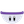#### Howdy, Stranger!

It looks like you're new here. If you want to get involved, click one of these buttons!

# Wrong answers when using trig functions

Posts: 524Memberedited November -1
When I try to verify equations, I find that trigonometric/circular functions such as cos and sin seem a bit wonky.

cos(137) = 0.73
sin(282) = 0.98

Using a calculator, I get these results:

cos(137) = 0.33
sin(282) = -0.68

What am I missing here? Can someone verify these answer?

Should I be using angle-type numbers instead of integers?

(It's probably something simple that I overlooked.)
This discussion has been closed.